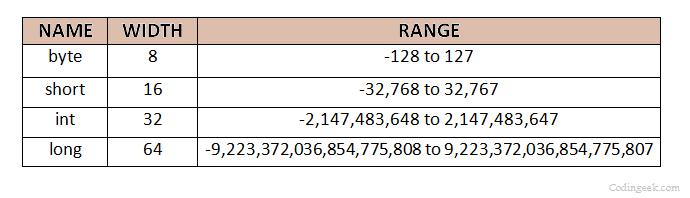# Primitive Data Types in Java-Integers, Floating Point, Character and Boolean

Java is strict in terms of type matches. Every variable or expression in Java must have a type which is defined in the Java Compiler. All explicit assignments or value assignments that are done using the method parameter must be in accordance with the types specified. So, in this tutorial, we shall see the different primitive data types and how to handle them.

There are eight primitive data types that are defined in Java, namely, byte, short, int, long, char, float, double and boolean. These can further be categorized into four groups:

• Integers: byte, short, int, and long fall under this category.
• Characters: This group contains char or a single character which can be any alphabetical letter or digit.
• Floating-point numbers: This group includes float and double which can be any fractional number.
• Boolean: The boolean data type falls under this category and represents true or false values.

These primitives or primitive data types are used to construct the reference data types like arrays in Java. An important point to be considered is that these data types have fixed values regardless of the environment or platform where it is executed. This is done to make Java a portable language(platform independent).

### Integers

There are four integer types in Java-byte, short, int and long. All of these are signed integers,i.e, it has positive as well as negative values. Java does not support unsigned (only positive) integers. Let’s get into more details about these four integer types:

• Byte: It has the least size range and is mostly used when working with the data stream from a network or file. Also, it is used when working with raw binary data.
Declaration example: byte a;
• Short: It is bigger than byte but shorter than int and long. It can be used when dealing with small numeric values.
Declaration example: short a;
• Int: It is the most commonly used integer data type which is bigger than a byte and short but smaller than long.
Declaration example: int a;
• Long: It is the largest integer data type and is useful when dealing with big, whole numbers.
Declaration example: long a;

The table below shows size in bits and range of the different integer data types in Java:Note that the default value for all the integer type data is 0 (zero).

### Characters

Java uses Unicode to represent characters which include a lot of character sets made up of languages such as Latin, Greek, Arabic, Katakana, etc. Since Unicode required 16 bits, the size of char in Java is 16 bits and the range is from 0 to 65,536. No negative char is required. The ASCII standard character set has the range 0 to 127 and the extended ISO-Latin-1 8-bit character set ranges from 0 to 255. But, the use of Unicode makes the language globally portable.

Declaration example: char ch=’x’;

char can also be used to store integer digits and can also be used to perform arithmetic operations. For example, ch++ is a valid statement in Java which increments the  ASCII(Unicode) value of the character stored and ‘y’ is stored in ch.

### Floating-Point Numbers

Floating-point number or the real number is used where the user requires fractional precision like square roots. Java uses the standard IEEE-754 set of floating-point types. The two type of Floating-point numbers in Java are:

• Float: Float type number have single precision and is faster on some processors due to less storage size, although it might become imprecise when dealing with very large or very small numbers.
Declaration example:
float a;
float b=10.9f;
In the case of initializing float variables the value must be followed by a letter f.
• Double: Double type number has double precision and is faster on modern processors and is optimized for high-speed mathematical calculations. It is useful in cases where we need high accuracy or when we have to deal with large-valued numbers. All transcendental mathematical functions like sin(), cos(), etc return double values in Java.
Declaration example:
double a;

The table below shows size in bits and range of the different floating-point data types in Java:The default value for floating-point numbers is 0.0.

### boolean

boolean is another primitive data type in Java which deals with logical values- true or false. All relational operators return a boolean value. This boolean value is used to control the if statement and the conditional loop statement. It can have either of the two values, i.e., either true or false.

Declaration example: boolean a, b = true;

Note – Remember that boolean(with small b) is a primitive type and is never null. It’s default value is false i.e. in the example above value of ‘a’ will be ‘false’. But there is also a class named Boolean(with capital B) which is an object and the Wrapper class of the primitive boolean.

So that’s all for this tutorial. Hope this helps and you like the tutorial. Do ask for any queries in the comment box and provide your valuable feedback. Do come back for more because learning paves way for a better understanding.

Keep Coding!! Happy Coding!! 🙂